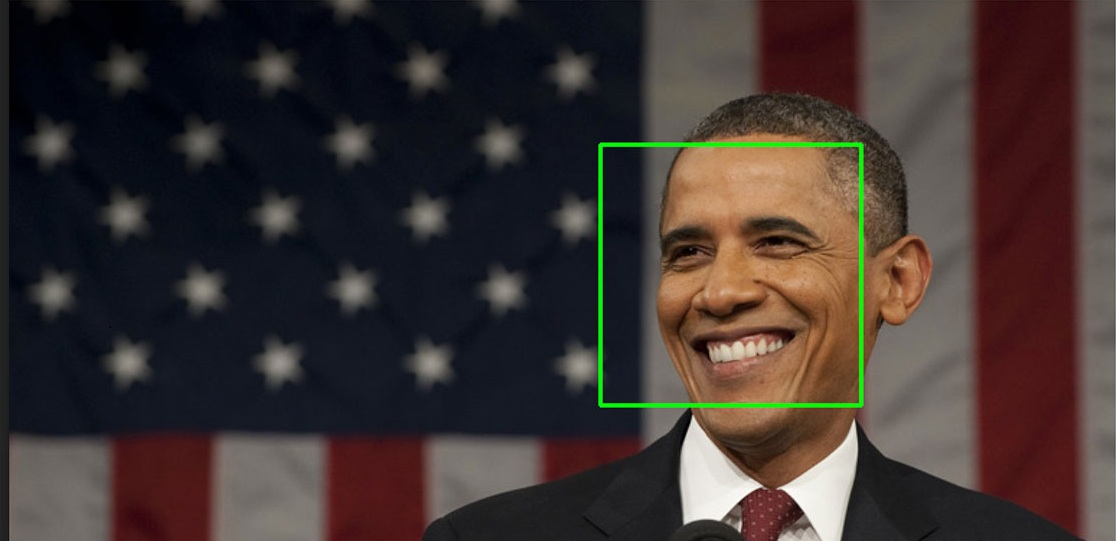/  Technology   /  Face Detection from an Image using OpenCV & Python## Face Detection from an Image using OpenCV & Python

#### OpenCV

OpenCV (Open Source Computer Vision Library) is an open-source computer vision and machine learning software library. This library mainly aims at real-time computer vision. In other words, we can say it is a library used for Image Processing.

It is mainly used to do all the operations related to Images like to analyze the data from the Camera of an Embedded system or your computer or anything that captures images (e.g. From a LIDAR).

It is written in C++ and supported by various programming languages such as C++, Java, Python. Some applications of OpenCV includes read and write Images, detection of faces and its features, text recognition in images, developing augmented reality apps.

First, let’s discuss

Computers read the image in the form of a matrix range between 0 to 255. For colored image, there are 3 channels Red, Green, Blue and there is a matrix associated with each of the channel, each element of this matrix represents the intensity of brightness of that pixel.

All the 3 channels will have their separate matrix which is stacked on each other to create 3-D metrics. For Gray/black image there is a 2-D matrix.

Coloured Image has 3 channels

Gray/Black scale image has 1 channel.

In the same way, the computer captures video. Video is multiple images/frames which are displayed very quickly.

Haar Cascade Classifier is a machine learning algorithm that is used to identify objects in images or videos. For detection of the image, the computer extracts features of an image and use those features to classify the image. It is an algorithm that works by classification.

The algorithm is fed a large number of images, positive (images with faces/objects we are interested in), and negative (images other than our target).

Then the algorithm extracts features from each image and classifies them into two parts. So depending upon the features extracted by the algorithm we use it to detect the object. Using Haar Cascade Classifiers you can detect the face of a person, eyes, objects such as cars, etc.

#### Installing OpenCV

For getting started with face detection we first need to install OpenCV library, you can install it by using

``pip install OpenCV-python``

if you need only main modules

``pip install OpenCV-contrib-python ``

if you need both main and contrib modules.

Also, it is recommended to store these XML files in the data folder in the same working directory as the jupyter notebook/python file.

#### Face Detection

``````import numpy as np
import cv2
%matplotlib inline
import matplotlib.pyplot as plt
import time

plt.imshow(cv2.cvtColor(img, cv2.COLOR_BGR2RGB))

def detect_faces_show(fpath):
gray = cv2.cvtColor(img, cv2.COLOR_BGR2RGB)

gray,
scaleFactor=1.1,
minNeighbors=6,
minSize=(40, 40),
)
print("Found %d faces!" % len(faces))

# Draw a rectangle around the faces
for (x, y, w, h) in faces:
cv2.rectangle(img, (x, y), (x+w, y+h), (0, 255, 0), 4)
plt.imshow(cv2.cvtColor(img, cv2.COLOR_BGR2RGB))

detect_faces_show('images/apink.jpg')
``````

Output:

In the above code we created a detect_faces_show function which is the most important part of the code.

In this function we used detectMultiScale module of the classifier. It returns rectangle with coordinates(x,y,w,h) around the detected face.

Then we loop over all the coordinates it returned and draw rectangles around them using OpenCV. And finally we displayed the image with all the faces detected correctly.